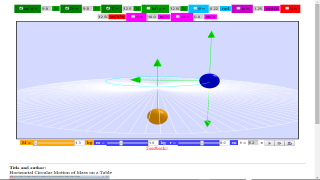Translations

Code Language Translator Runid Indonesian Bahasa IndonesiaSoftware Requirements

SoftwareRequirements

 Android iOS Windows MacOS with best with Chrome Chrome Chrome Chrome support full-screen? Yes. Chrome/Opera No. Firefox/ Samsung Internet Not yet Yes Yes cannot work on some mobile browser that don't understand JavaScript such as..... cannot work on Internet Explorer 9 and below

CreditsFu-Kwun Hwang - Dept. of Physics, National Taiwan Normal Univ. and lookang; lookang; tinatan

end faq

http://iwant2study.org/lookangejss/02_newtonianmechanics_6circle/ejss_model_circular3dfr02wee/circular3dfr02wee_Simulation.xhtml

Learning Content

Motion in a Circle Content taken from http://www.seab.gov.sg/content/syllabus/alevel/2017Syllabus/9749_2017.pdf

1. Kinematics of uniform circular motion
2. Centripetal acceleration
3. Centripetal force

Learning Outcomes

Candidates should be able to:

1. express angular displacement in radians
2. show an understanding of and use the concept of angular velocity to solve problems
3. recall and use v = rω to solve problems
4. describe qualitatively motion in a curved path due to a perpendicular force, and understand the centripetal acceleration in the case of uniform motion in a circle
5. recall and use centripetal acceleration a = rω 2 , and $$a = \frac{v^2 }{r}$$ to solve problems
6. recall and use centripetal force F = mrω 2 , and $$F = \frac{mv^2}{r}$$ to solve problems.

For Teachers

A particle with mass $$m$$ is moving with constant speed $$v$$ along a circular orbit (radius $$r$$). The centripetal force $$F=m\frac{v^2}{r}$$ is provided by gravitation force from another mass $$M=F/g$$.
A string is connected from mass m to the origin then connected to mass $$M$$.
Because the force is always in the $$\hat{r}$$ direction, so the angular momentum $$\vec{L}=m\,\vec{r}\times \vec{v}$$ is conserved. i.e. $$L=mr^2\omega$$ is a constant.

For particle with mass m:

$$m \frac{d^2r}{dt^2}=m\frac{dv}{dt}= m \frac{v^2}{r}-Mg=\frac{L^2}{mr^3}- Mg$$
$$\omega=\frac{L}{mr^2}$$

The following is a simulation of the above model.

When mass m or radius r is changed with sliders, equilibrium condition is recalulated for constant circular motion.
However, if mass M is changed, the equilibrium condition will be broken, and the system will oscilliate up and down.

[text]

Video

Ejs Open Source Horizontal Circular Motion java applet by lookang lawrence wee

Version:

1. http://weelookang.blogspot.sg/2016/03/horizontal-3d-webgl-circular-motion-of.html HTML5 JavaScript WebGL version by Loo Kang Wee and Tina Tan
2. http://weelookang.blogspot.sg/2010/07/lesson-on-circular-motion-with-acjc.html 09 July 2010 Computer Lab hands on learning session on  Ejs Open Source Vertical Circular Motion of mass m attached to a rod java applet side view of the same 3D view with teacher explaining the physical setup of the mass m and mass M attached by a string through a table with a fricitionless hole in the middle of table for string to go through and student working on their own desktop
3. http://www.phy.ntnu.edu.tw/ntnujava/index.php?topic=1883.0 remixed Java applet by Loo Kang Wee
4. http://www.phy.ntnu.edu.tw/ntnujava/index.php?topic=1454.0 original Java applet by Fu-Kwun Hwang

end faq